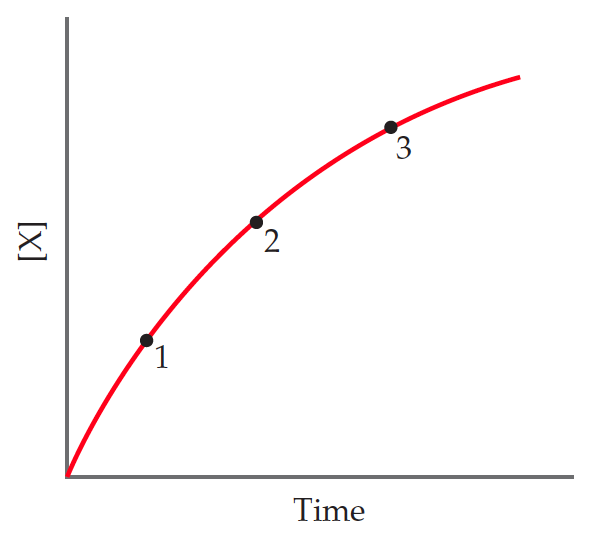# Problem: Consider the following graph of the concentration of a substance X over time.Is each of the following statements true or false?(a) X is a product of the reaction.(b) The rate of the reaction remains the same as time progresses.(c) The average rate between points 1 and 2 is greater than the average rate between points 1 and 3.(d) As time progresses, the curve will eventually turn downward toward the x-axis.

🤓 Based on our data, we think this question is relevant for Professor Wilson's class at Saint Louis Community College.

###### FREE Expert SolutionAnalyzing each statement:

(a) X is a product of the reaction.

• as time goes by the concentration of [X] increases

• X is being produced

The statement is TRUE.

(b) The rate of the reaction remains the same as time progresses.

• the progress of the reaction is not linear

• amount of X produced is not constant

The statement is FALSE.

(c) The average rate between points 1 and 2 is greater than the average rate between points 1 and 3.###### Problem Details

Consider the following graph of the concentration of a substance X over time.Is each of the following statements true or false?
(a) X is a product of the reaction.
(b) The rate of the reaction remains the same as time progresses.
(c) The average rate between points 1 and 2 is greater than the average rate between points 1 and 3.
(d) As time progresses, the curve will eventually turn downward toward the x-axis.